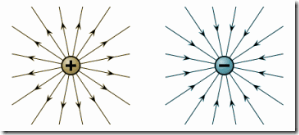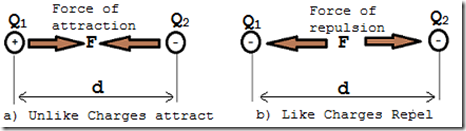# Laws of ElectrostaticsIn general an electrical engineering that deals with electricity at rest is termed electrostatics. All the electrical phenomena are created due to the varieties of charges. The moving charges create current and magnetic effects. The accelerated charges create radiation. With the exception of moving and accelerated charges, there exists an additional variety of charges referred to as stationary charges or static charges. Static charges are accountable for the generation of the forces on different charges that are referred to as electrostatic forces. Electrostatic means that the study of the static charges and also the associated effects.
The two basic laws of electrostatics are as below:

#### Like charges repel one another and unlike charges attract one another

The law will be validated by an easy experiment. The ebonite rod becomes negatively charged once rubbed against fur cloth. Now, if glass rod is rubbed against fur cloth, it gets positively charged. And if they're brought close to one another, they try to attract one another. Whereas 2 hard rubber (ebonite) rods once rubbing against fur cloth brought near, try to repel one another. This shows that like charges repel whereas unlike charges attract one another.

#### Coulomb's Inverse square Law

The law conditions that the mechanical force, attraction or repulsion, between the 2 little charged bodies is
• Directly proportional to the product of the charges present on the bodies.
• Inversely proportional to the sq. of the distance between the bodies and
• Depends upon the character of the medium nearby the bodies.The Fig. above shows 2 point charges, parted by distance ‘d’ meters. The charges are Q1 and Q2 coulombs and K is the constant of proportion. According to law of nature, force between the charges will be mathematically expressed as,
F ∝ (Q1Q2)/d2
F = (KQ1Q2)/d2 Newton's
The constant of proportionality, k depends in the nearby medium and is specified by,
K = 1/ (4πϵ) = 1 / (4π ϵr ϵ0)
Where,
ϵ = Absolute permittivity of the medium = ϵr ϵ0
ϵo = Permittivity of free space
ϵr = Relative permittivity of the medium
ϵ0 = 1 / (36π x 109)
For air,
ϵr = 1
For example If Q1=Q2= 1 C and d = 1m
Then    F = 1/(4x π x 8.854x10-12) = 9x109 N0 CommentsComments